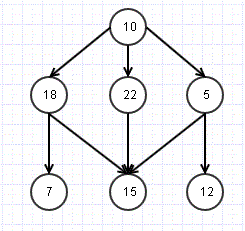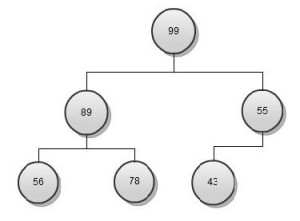# Depth First Search

In last post I talked about Breadth First Search. An alternative to that, is Depth First Search, used in cases we want to check upto a depth level first rather than breadth.

So let’s look back at the last exampleThis time we would like to parse something like

1. Check Root- 10

2. Check till depth level for one node- 18,7

3. Repeat for other nodes 22,15 and then 5, 12

A straight forward way is to modify the Breadth First Search algorithm to use Stack instead of Queue.

1. Push Root Node R to Stack S
2. Pop s node from S
3 a . If s.val is required value, return Success
3 b. Else Push all Neighbor/ child nodes to stack
4. If Stack is not empty, Repeat from 2
5. If Stack is empty, we have traversed all the nodes and not found the required node. Return failure.

# Breadth First Search

Breadth First search is an effective search mechanism for Graphs and Tree Data structure. As the name suggest, this technique makes sure that all the nodes at same level/ breadth are checked before moving to deeper levels.The above structure is hybrid of tree and graph (Still a tree as there are no cycles). Let us what it means to do a breadth first search here.

We will start with root node i.e. 10.

Move to level 2 and check nodes 18, 22, 5.

Check the next level- 7, 15, 12 and so on.

Here is algorithm to implement Breadth first search

1. Add root node R to queue- Q

2. Fetch next node N from Q

3 a. If the N.val is required val, return success

3 b. Else Find all Child/ Neighbour nodes which are not traversed already and add to Queue

4. If there are more nodes in Q, Repeat from 2.

5. If Q is empty, we know we have traversed all nodes, return failure as node not found

Read more here- http://en.wikipedia.org/wiki/Breadth-first_search

http://en.wikipedia.org/wiki/Breadth-first_search

# Quick Sort: Algorithm and Complexity

After talking about Insertion sort and Heap sort, another important sorting algorithm is quick sort. It is pretty simple algorithm, and will be easy to understand using an example. Lets say we have this random array which we are trying to sort

9, 5, 7, 1, 2, 4, 3, 8, 6

Most critical step of quick sort is to select a pivot element from the array. There are multiple approaches to select the pivot like- first element, last element, mid element, random element, mean, median etc. For sake of simplicity, lets say we randomly select the pivot as 5 for first iteration. Once pivot is selected, the array is divided into 2 arrays, one with elements smaller than pivot and other greater

Iteration 1:

A1: 1, 2, 4, 3

P: 5

A2: 9, 7, 8, 6

In next iteration, one of the arrays (A1) is chosen and again a pivot (say 3) is selected. The same exercise is repeated of breaking down the array into 2 sub arrays unless we get an array of size 1. After that the arrays are merged recursively in same order as they were created, ending up into final sorted array.

Average & Best Case: If we take above case where we kept on dividing the problem array into half (N/2 then N/4, N/8 and so on), and hence halfing number of comparison in every next step. we are looking at a complexity of N Log N.

Worst Case: In worst case, lets say we select highest element every time. So instead of having half the elements in an array, we have N-1 elements in next step. So in this case we end up N*N (N Square) complexity

# Heap Data Structure

Understanding of Heap data structure is very important as it is part of many other data structures like priority queue and algorithms like priority queues, Dijkstra’s.

Let’s understand Heap data structure first. Heap is a simple tree based data structure with a unique property that root node is always larger or smaller than its children.In the current heap we can see parent element is always higher than child nodes, hence it is called max heap. Similarly in case the parent is smaller than children, it will be min heap. Also the heap shown above is binary heap as each parent node has only two child nodes.

Let’s talk about efficiency of the heap data structure in terms of Inserting and deleting the nodes.

Insert a Key: To insert a new node, new element is added to next possible leaf node. It is then compared to its parent node and moved up if larger.

1. Insert node at next leaf

2. While element is less than parent

Swap child and parent

Insert operation in a heap data structure is order of log N, where N is number of nodes available.

Delete Node: Deletion always occur at the root node. Last leaf node is moved to root and then moved down till it is greater than children.

1. Node =root

2. While node <any child

Swap node and largest child

Delete operation in a heap data structure is order of log N, where N is number of nodes available.